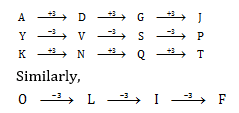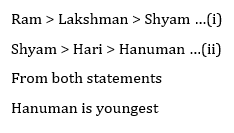# RRB JE General intelligence & Reasoning quiz for (Phase I) and other railways exams 30/01/2019

General intelligence & Reasoning quiz for RRB JE (Phase I), RRB NTPC, SSC various exams and other competitive exams.

Q1,.A statement with two conclusions are given followed by four alternatives. Select the one which is most appropriate.

Statements :
No man is a donkey.
Rahul is a man.
Conclusions:
I. Rahul is not a donkey
II. All men are not Rahul.

A) Only I

B) Only II

C) Either I or II

D) Neither I nor II

Q2. In the following question, select the related words/letters numbers from the given alternatives.

4 : 17 : : 7 : ?

A) 50

B) 48

C) 49

D) 51

Q3. In the following question, select the odd one from the given alternatives.

A) 576 – 12

B) 611 – 13

C )198 – 11

D) 824 – 14

Q4. In the following question, select the odd one from the given alternatives.

A) Snooker

B) Table Tennis

D) Billiards

Q5. Arrange the following words according to dictionary sequence.

1.Brother

Husband

3.Father

4.Son

5.Son – in – law

A) 3, 2, 1, 5, 4

B) 4, 1, 2, 5, 3

C) 3, 1, 4, 2, 5

D) 1, 3, 2, 4, 5

Q6. In each of the following, a series is given with one term missing. Choose the correct alternative from the given ones that will complete the series.

A) SVZB

B) QTWZ

C)  LORU

D) OLIF

Q7. Select the correct combination of mathematical signs to replace * signs and to balance the given equation.

2 * 4 * 3 * 4 * 9

A) + × = –

B) × ÷ – =

C) × – + =

D) + – = ÷

Q8. If PKROK is coded as 72962 and KRRPK as 29972 then how can NJMLZ be coded ?

A) 51438

B) 45176

C) 74314

D) 91572

Q9. Lakshman is elder than Shyam, but younger than Ram. Hanuman is younger than both Hari and Shyam. Shyam is elder than Hari. Who is the youngest?

A) Ram

B) Laksham

C) Hanuman

D) Hari

Q10. In each of the following questions, select the missing number from the given responses.

A) 26

B) 30

C) 25

D) 23

# Solutions

Q1. Ans(A)

Since, on Premise is negative, the conclusion must be negative. Conclusion II cannot follow as it contains the middle term. So, only I follows.

Q2. Ans(A)

Pattern is – a : a2 + 1

Q3. Ans(D)

Except in the number pair 824 – 14, in all others the first number is completely divisible by the second number.

Q4. Ans(C)

Except Badminton, all other games are played on tables, Badminton is played in court

Q5. Ans(D)

Q6. Ans(D)Q7 Ans(C)

2× 4 – 3 + 4 = 12 – 3 = 9

Q8. Ans(A)Q9. Ans(C)Q10. Ans(C)

The sum of all the three numbers in each column is 81.

## WhatsApp Group Join here

Mail us at : ambitiousbaba1@gmail.com

3